## Quickly add hours/minutes/seconds to datetimes in Excel

Managing dates and times in Excel can often be a meticulous task, especially when you need to modify time across numerous cells. Whether you're adjusting project timelines, scheduling events, or logging data, adding specific time increments to datetimes is a common necessity. In this article, we'll explore how to quickly add hours, minutes, and seconds to the mixed datetime cells in Excel.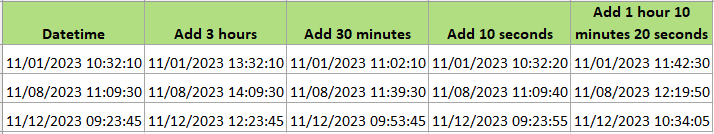### Using formulas to add hours/minutes/seconds to datetime

This section outlines four distinct formulas to help you efficiently add a specific number of hours, minutes, and seconds to datetime values in Excel, either separately or as a combined time value. Follow these steps to apply each formula effectively:

##### Step 2: Using formula to add hours, minutes and seconds

In the selected blank cell , please use one of below formulas as you need.

To add a specific number of hours to a datetime in Excel, you can use a straightforward formula.

The general formula is:

datetime + num_hours/24

Here’s how you can apply this:

Example: To add 3 hours to a datetime in cell A2, use the formula:

``=A2 + 3/24``

This formula works because there are 24 hours in a day, so dividing the number of hours by 24 converts it into the equivalent fraction of a day. Adding this fraction to the original datetime results in the datetime being increased by the specified number of hours.

To add a specific number of minutes to a datetime in Excel, you can use a straightforward formula.

The general formula is:

datetime + num_minutes/1440

Here’s how you can apply this:

Example: To add 30 minutes to a datetime in cell A2, use the formula:

``=A2 + 30/1440``

This formula works because there are 1440 minutes in a day, so dividing the number of minutes by 1440 converts it into the equivalent fraction of a day. Adding this fraction to the original datetime results in the datetime being increased by the specified number of minutes.

To add a specific number of seconds to a datetime in Excel, you can use a straightforward formula.

The general formula is:

datetime + num_seconds/86400

Here’s how you can apply this:

Example: To add 10 seconds to a datetime in cell A2, use the formula:

``=A2 + 10/86400``

This formula works because there are 86400 minutes in a day, so dividing the number of seconds by 86400 converts it into the equivalent fraction of a day. Adding this fraction to the original datetime results in the datetime being increased by the specified number of seconds.

• Add hours, minutes and seconds to datetime

To add mixed value of hours, minutes and seconds to a datetime in Excel, you can use the TIME function.

The general formula is:

Datetime + TIME(num_hours, num_minutes, num_seconds)

Example: To add 1 hour, 10 minutes and 20 seconds to a datetime in cell A2, use the formula:

``=A2 + TIME(1,10,20)``

Then press Enter key, and drag auto fill handle over the cells to apply the formula if you need.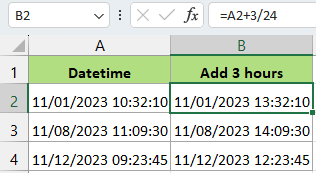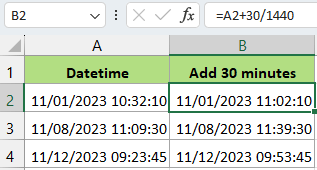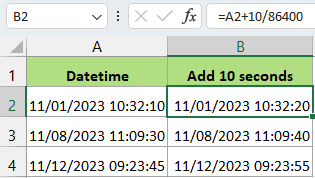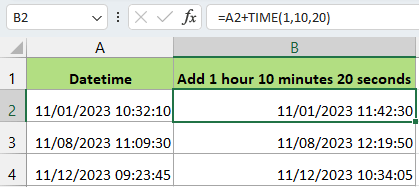Tip: For subtracting time from datetimes, just change the plus sigh (+) to minus sign (-).
##### (Optional) Step 3: Format cells

If the results of your calculation are displayed in a numerical format rather than as datetime, you can easily change this with a few quick steps:

1. Select the Results: Click and drag to highlight the cells that contain your calculation results.
2. Open Format Cells Dialog: Either press Ctrl + 1 on your keyboard, or right-click on the selected cells and choose Format Cells from the context menu.
3. Set the Correct Format:
1. Under Number tab, choose Custom from the Categories list,
2. Type mm/dd/yyyy hh:mm:ss in the Type textbox, (Or type other formats as you need,)
3. Click OK.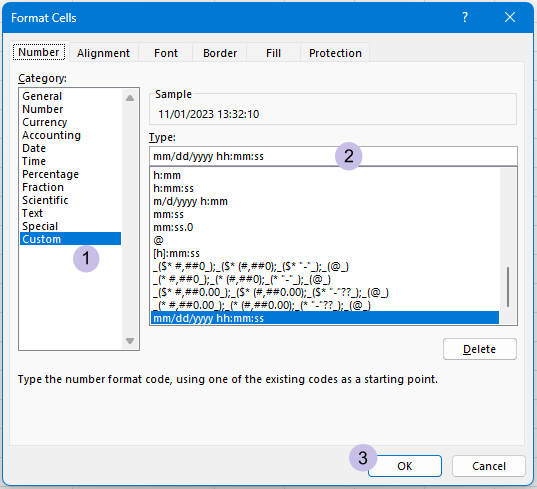By applying these steps, you'll convert the numerical output back into a readable datetime format, ensuring your data is both accurate and clearly presented.

#### Make Datetime Calculation Fly

Kutools for Excel's Date Time Helper is an incredibly efficient tool designed to simplify complex date and time calculations. Give it a try and see how it transforms your data management experience!

• ##### Quickly add or subtract time to datetime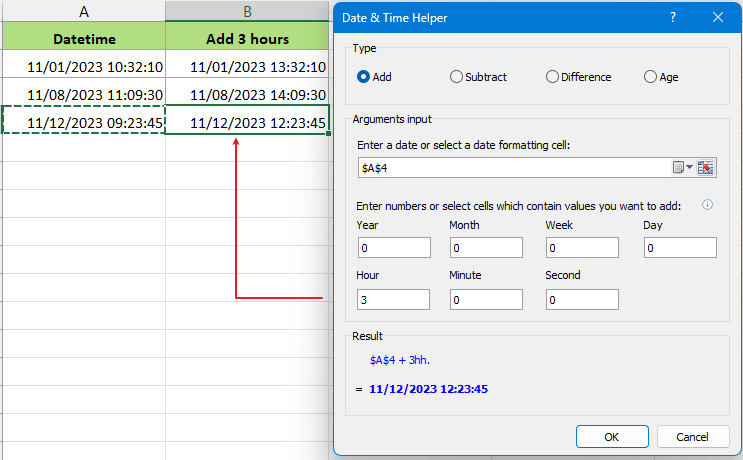• ##### Easily calculate difference between two datetimes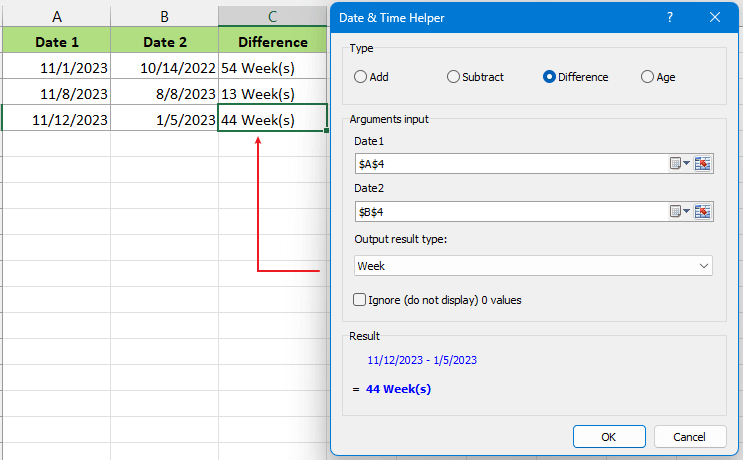• ##### Efficiently Calculate Age from a Date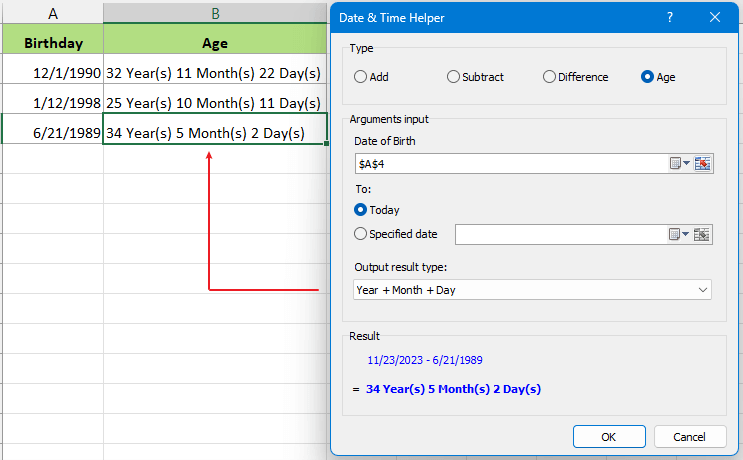### Using a handy tool to add hours/minutes/seconds to datetime

Kutools for Excel's Date Time Helper is a game-changer for managing dates and times in your spreadsheets. This tool simplifies complex tasks like adding or subtracting time, calculating age, and managing intervals, all without the need for complex formulas. Its intuitive interface integrates smoothly with Excel, making it perfect for both beginners and power users.

Install Kutools for Excel: Download and install Kutools for Excel for a wide range of convenient Excel functions.

Select a blank cell that will output the result, then click Kutools > Formula Helper > Date & Time helper, in the popping dialog, please:

2. Click the select button to pick a cell with the datetime you're modifying.
3. Enter the hours, minutes, and seconds to add.
4. Preview the result in the Result section and click OK.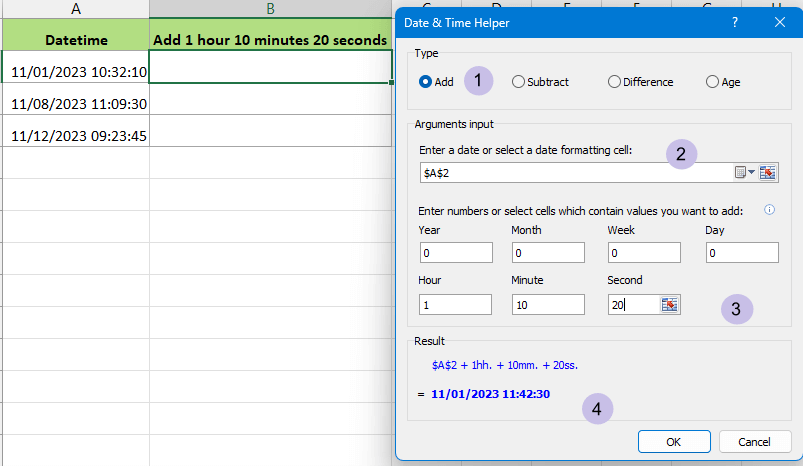Then use the autofill handle to apply this adjustment to other cells as needed.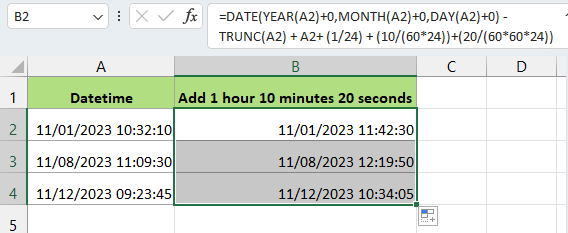Both methods, whether the traditional formula approach or the streamlined Kutools feature, can significantly reduce the time spent on modifying datetime data in Excel. Choosing the right method depends on your specific needs and the complexity of your data. For more Excel insights and tips, continue exploring our articles, designed to enhance your productivity and data management skills.

### The Best Office Productivity Tools

#### Kutools for Excel - Helps You To Stand Out From Crowd

 Popular Features: Find, Highlight or Identify Duplicates  |  Delete Blank Rows  |  Combine Columns or Cells without Losing Data  |  Round without Formula ... Super VLookup: Multiple Criteria  |  Multiple Value  |  Across Multi-Sheets  |  Fuzzy Lookup... Adv. Drop-down List: Easy Drop Down List  |  Dependent Drop Down List  |  Multi-select Drop Down List... Column Manager: Add a Specific Number of Columns  |  Move Columns  |  Toggle Visibility Status of Hidden Columns  |  Compare Columns to Select Same & Different Cells ... Featured Features: Grid Focus  |  Design View  |  Big Formula Bar  |  Workbook & Sheet Manager | Resource Library (Auto Text)  |  Date Picker  |  Combine Worksheets  |  Encrypt/Decrypt Cells  |  Send Emails by List  |  Super Filter  |  Special Filter (filter bold/italic/strikethrough...) ... Top 15 Toolsets:  12 Text Tools (Add Text, Remove Characters ...)  |  50+ Chart Types (Gantt Chart ...)  |  40+ Practical Formulas (Calculate age based on birthday ...)  |  19 Insertion Tools (Insert QR Code, Insert Picture from Path ...)  |  12 Conversion Tools (Numbers to Words, Currency Conversion ...)  |  7 Merge & Split Tools (Advanced Combine Rows, Split Excel Cells ...)  |  ... and more

Kutools for Excel Boasts Over 300 Features, Ensuring That What You Need is Just A Click Away...#### Office Tab - Enable Tabbed Reading and Editing in Microsoft Office (include Excel)

• One second to switch between dozens of open documents!
• Reduce hundreds of mouse clicks for you every day, say goodbye to mouse hand.
• Increases your productivity by 50% when viewing and editing multiple documents.
• Brings Efficient Tabs to Office (include Excel), Just Like Chrome, Edge and Firefox.No ratings yet. Be the first to rate!
This comment was minimized by the moderator on the site
Hi,
I have a register of miliseconds in a raw base data, but I need to have these miliseconds in a format of mm/ss, how can I change it? Is there any kind of formula?
This comment was minimized by the moderator on the site
This comment was minimized by the moderator on the site
Hi, Sn mishra, to add the fixed time 24 hours to a date, why you not directly add one day to the date? In this case, you can use the formula date+1 to get the result, such as ="2/3/2021 2:10"+1
This comment was minimized by the moderator on the site
How about subtracting 15 hours from a time? I have a worksheet with releases from river headgates and need to put in a formula to tell client what time to release water into river (15 hours prior to 8 AM).
This comment was minimized by the moderator on the site
Hi, Jerry, to subtract 15 hours from a time, just use the formula time-15/24 to get the result.
This comment was minimized by the moderator on the site
I know this is an old thread, but this doesn't work for me using KuTools. Does the original data have to be in a certain format? I've tried both general and custom (mm-dd-yyyy hh:mm:ss). Any help would be greatly appreciated!
This comment was minimized by the moderator on the site
This is really helpful. Thanks a lot! :-)
This comment was minimized by the moderator on the site
Thank you very much for this.
This comment was minimized by the moderator on the site
it works well, thanks...
This comment was minimized by the moderator on the site
looks nice, but not working, what version of excel are you presenting?
This comment was minimized by the moderator on the site
NICE IT WORKS THANK U
This comment was minimized by the moderator on the site
this add in does not even work. I tried the exact example above and I just get errors.
This comment was minimized by the moderator on the site
Could you give me a specific example?
This comment was minimized by the moderator on the site
Thank u for ur remind.! I have corrected it. Thanks again.
There are no comments posted here yet